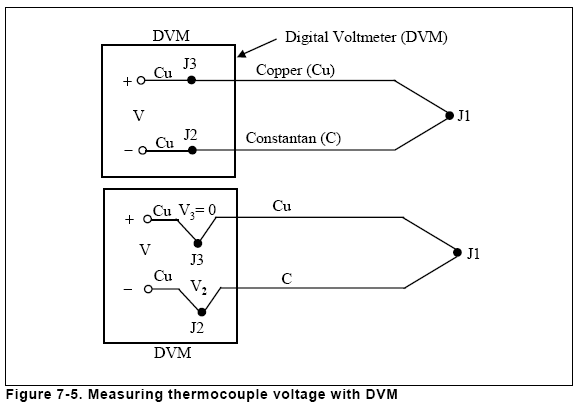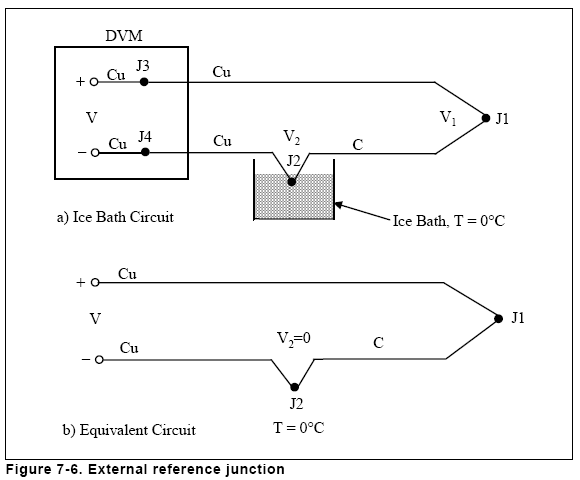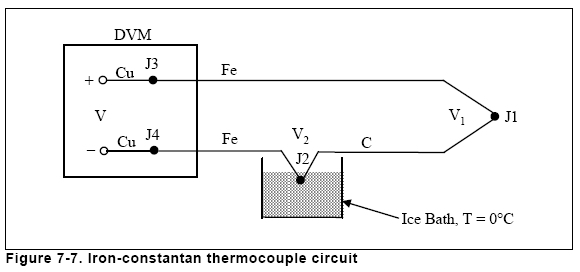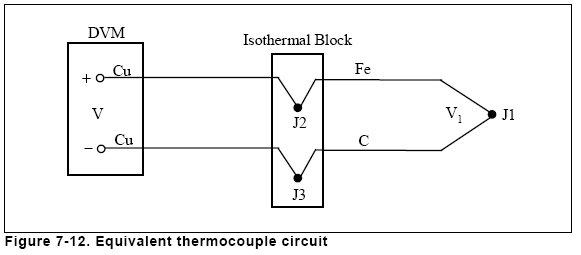Measurement and Control Basics Fourth Edition

# Chapter 7 - Temperature Measurement: Measuring Thermocouple Voltage

You cannot measure the Seebeck voltage directly because you must first
connect a voltmeter to the thermocouple, and the voltmeter leads create a
new thermoelectric circuit.

Consider a digital voltmeter (DVM) that is connected across a copper-constantan
(type T) thermocouple. The voltage output is shown in Figure 7-5.We would like the voltmeter to read only V1, but to measure the output of
junction J1 we have connected the voltmeter, which created two more
metallic junctions: J2 and J3. Since J3 is a copper-to-copper junction, it creates
no thermal voltage (V2 = 0). However, J2 is a copper-to-constantan
junction, which will add a voltage V2 in opposition to V1. The resultant
voltmeter reading V will be proportional to the temperature difference
between J1 and J2. This indicates that we cannot find the temperature at J1
unless we first find the temperature of J2.

One way to determine the temperature of J2 is to physically put the junction
into an ice bath. This forces its temperature to be 0°C and establishes
J2 as the "reference junction," as shown in Figure 7-6. Since both voltmeter
terminal junctions are now copper-copper, they create no thermal voltage,
and the reading "V" on the voltmeter is proportional to the temperature
difference between J1 and J2.Now the voltmeter reading is as follows:If we specify Tj1 in degrees Celsius,then V becomes the following:We presented this somewhat involved discussion to emphasize that the
ice-bath junction output, V2, is not zero volts. It is a function of absolute
temperature.

By adding the voltage of the ice-point reference junction, we have now referenced
the TC voltage reading (V) to 0°C. This method is very accurate
because the ice-point temperature can be precisely controlled. The ice
point is used by the National Bureau of Standards (NBS) as the fundamental
reference point for their thermocouple tables. Now we can look at the
thermocouple tables in Appendix B and directly convert from voltage V
into temperature.

The copper-constantan thermocouple circuit shown in Figure 7-6 is a
unique example because the copper wire is the same metal as the voltmeter
terminals. Consider an iron-constantan thermocouple (type J) instead
of the copper-constantan (Figure 7-7). The iron wire increases the number
of dissimilar metal junctions in the circuit, since both voltmeter terminals
become Cu-Fe thermocouple junctions.This circuit will provide moderately accurate measurements as long as the
voltmeter (positive) and (negative) terminals (J3 and J4) are the same temperature.
The thermoelectric effects of J3 and J4 act in opposition,

 V1 = V

if,

 V3 = V4

that is, if

 Tj3 = Tj4

If both front-panel terminals are not at the same temperature, an error will
result. To gain a more precise measurement, you should extend the copper
(same temperature) block, as shown in Figure 7-8.The isothermal block is not only a good electrical insulator. It is also a
good heat conductor, and this helps to hold J3 and J4 at the same temperature.
The absolute block temperature is unimportant because the two
Cu-Fe junctions act in opposition. Thus,The circuit in Figure 7-8 will provide accurate readings, but it is desirable
to eliminate the ice bath if possible. One way to do this is to replace the ice
bath with another isothermal block, as shown in Figure 7-9.The new block is at reference temperature Tref, and because J3 and J4 are
still at the same temperature we can again show thatThis is still a complicated circuit because we have to connect two thermocouples.
Let's eliminate the extra Fe wire in the negative (-) lead by combining
the Cu-Fe junction (J4) and the Fe-C junction (Jref). We can do this by
first joining the two isothermal blocks (Figure 7-10). We have not changed
the output voltage V. It is still as follows:Now we can use the law of intermediate metals to eliminate the extra junction.
This empirical "law" states that a third metal (in this case, iron)
inserted between the two dissimilar metals of a thermocouple junction
will have no effect on the output voltage as long as the two junctions
formed by the additional metal are at the same temperature (see
Figure 7-11).This is a useful result, as it eliminates the need for the iron wire in the negative
lead. This can be seen in Figure 7-12. There again, V = a (T1 - Tref),
where a is the Seebeck coefficient for a Fe-C thermocouple. Junctions J2
and J3 take the place of the ice bath. These two junctions now become the
"reference junction."Now we can proceed to the next logical step: directly measure the temperature
of the isothermal block (the reference junction) and use that information
to compute the unknown temperature, Tj1. The thermistor is a
temperature-measuring device whose resistance Rt is a function of temperature.
It provides us with a method for measuring the temperature of
the reference junction (Figure 7-13). We assume that the junctions J2 and J3
and the thermistor are all at the same temperature because of the design of
the isothermal block. Using a digital voltmeter under computer control,
we simply do the following:

1. Measure Rt to find Tref, and convert Tref to its equivalent reference
junction voltage, Vref.
2. Measure V and subtract Vref to find V1, and convert V1 to
temperature Tj1.This procedure is known as "software compensation" because it relies on
a computer's software to compensate for the effect of the reference junction.
The isothermal terminal block temperature sensor can be any device
that has a characteristic that is proportional to absolute temperature: a
resistance temperature detector (RTD), a thermistor, or an integrated-circuit
sensor.

The logical question is, "If we already have a device that will measure
absolute temperature (such as an RTD or thermistor), why do we even
bother with a thermocouple that requires reference junction compensation?"
The single most important answer to this question is that the thermistor,
the RTD, and the integrated-circuit transducer are useful only over
a limited temperature range. You can use thermocouples, on the other
hand, over a wide range of temperatures. Moreover, they are much more
rugged than thermistors (as evidenced by the fact that thermocouples are
often welded to metal process equipment or clamped under a screw on the
equipment). They can be manufactured easily, either by soldering or
welding.

In short, thermocouples are the most versatile temperature transducers
available. Furthermore, a computer-based temperature-monitoring system
can perform the entire task of reference compensation and software
voltage-to-temperature conversion. As a result, using a thermocouple in
process control becomes as easy as connecting a pair of wires. The one disadvantage
of the computer-based approach is that the computer requires a
small amount of extra time to calculate the reference junction temperature,
and this introduces a small dead time into a control loop.### This is embarrasing...

An error occurred while processing the form. Please try again in a few minutes.